Question

1 Consider the system shown as below. Draw a Bode diagram of the open-loop transfer function G(s). Determine the phase margin, gain-crossover frequency, gain margin and phase-crossover frequency, (Sketch the bode diagram by hand)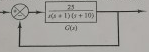2 Consider the system shown as below. Use MATLAB to draw a bode diagram of the open-loop transfer function G(s). Show the gain-crossover frequency and phase-crossover frequency in the Bode diagram and determine the phase margin and gain margin.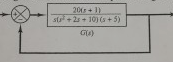3. Consider the system shown as below. Design a lead compensator such that the dominant closed-loop poles are located at s=2±j3.464. Plot the unit-step and nit-ramp response curves of the compensated system with MATLAB.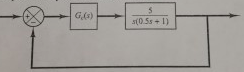4. Referring to the closed-loop system shown as below, design a lead compensator Ge(s) such that the phase-margin is 45°, gain margin is not less than 8dB, and the static velocity error constant K, is 4.0 sec-1. Plot unit-step and unit-ramp response curves of the compensated system with MATLAB.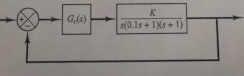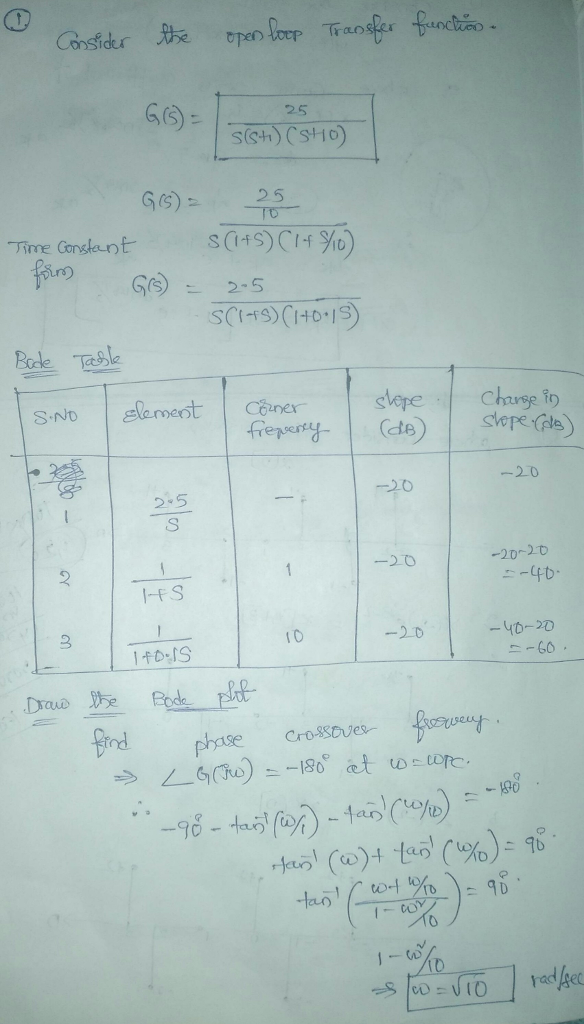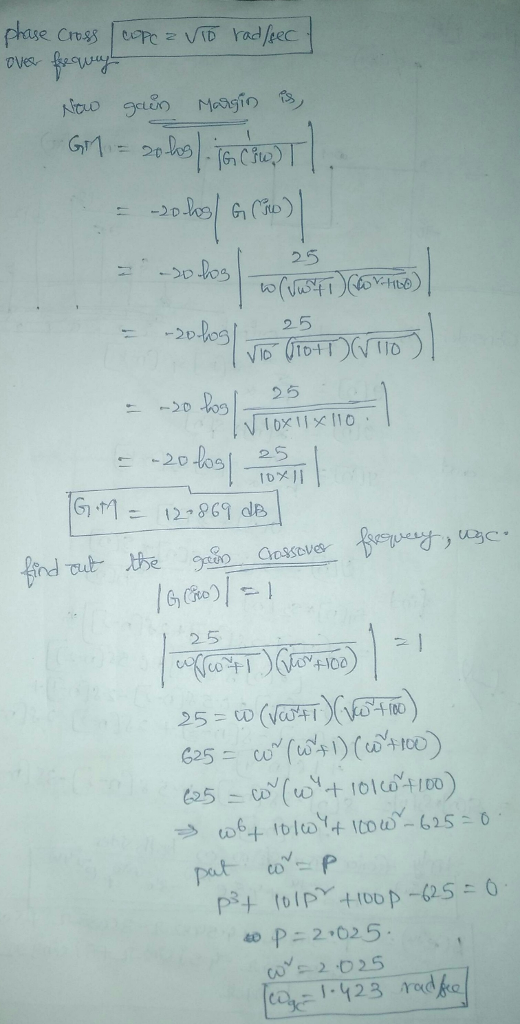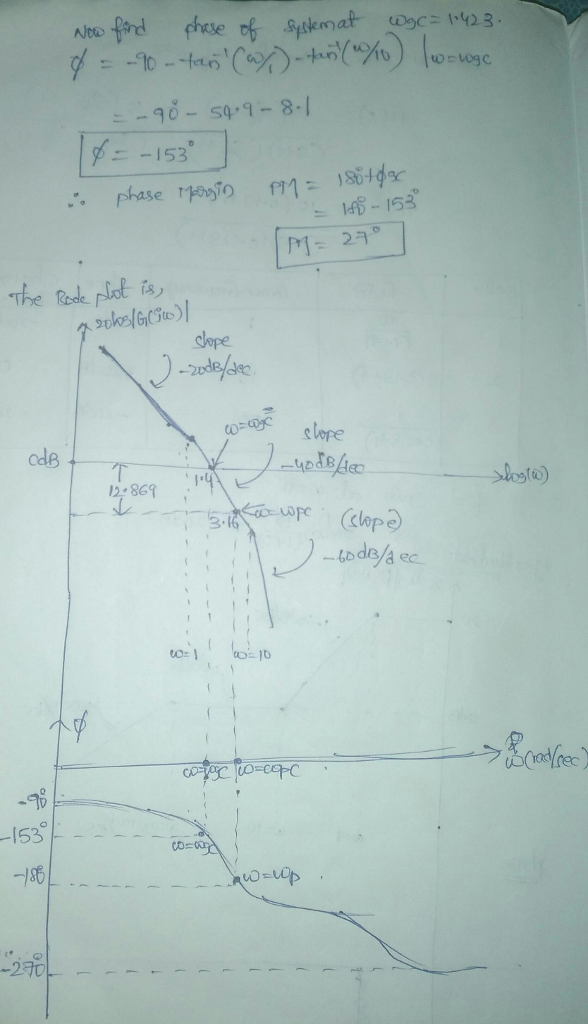#### Earn Coins

Coins can be redeemed for fabulous gifts.

Similar Homework Help Questions
• ### 4. Referring to the closed-loop system shown as below, design a lead compensator Ge(s) such that...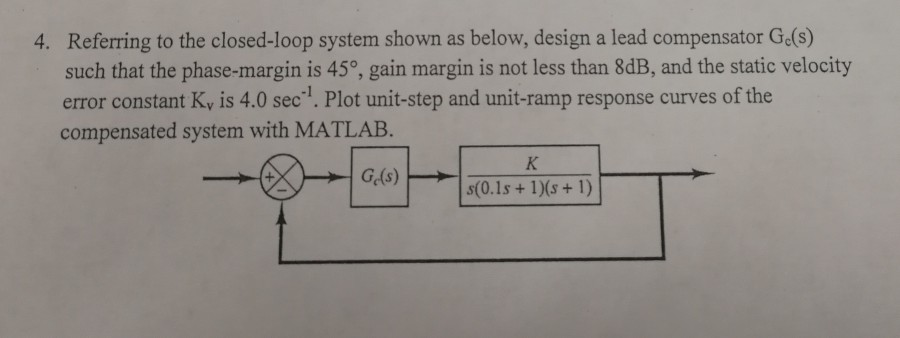4. Referring to the closed-loop system shown as below, design a lead compensator Ge(s) such that the phase-margin is 45o, gain margin is not less than 8dB, and the static velocity error constant Ky is 4.0 sec1. Plot unit-step and unit-ramp response curves of the compensated system with MATLAB.

• ### Bode Diagram 10 10 Frequency (rad/s) Bode Diagram 100F 140 10 10 Frequency (rad/s) Figure Q4.2 4. The de servo system s...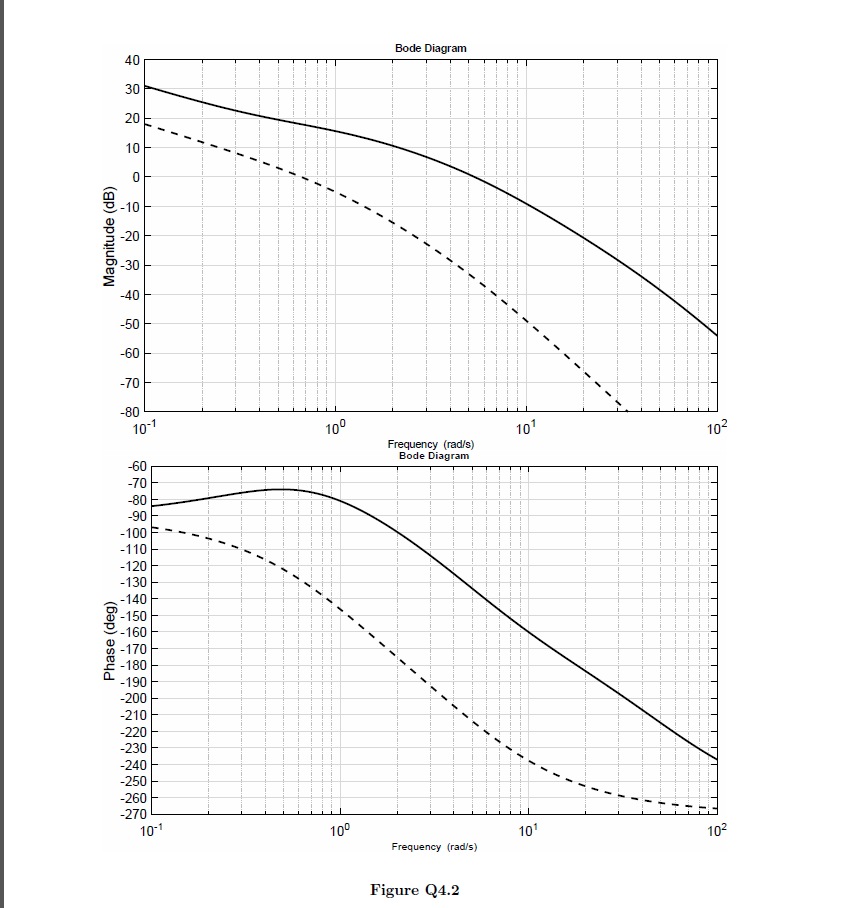Bode Diagram 10 10 Frequency (rad/s) Bode Diagram 100F 140 10 10 Frequency (rad/s) Figure Q4.2 4. The de servo system shown in Figure Q4.1 is required to have a transient step response speci fication with a peak time of 0.58 seconds or better, and a +2% setting time of 1.7 seconds or better 01(s) K (s)G(s) s(s 1 (s 5) Figure Q4.1 The Bode diagram of the open-loop system is shown in Figure Q4.2 on page 8. This Bode...

• ### Y(s) C(s) G(s) R(S) Figure 1: Closed-loop system Q2 Consider the setup in Figure 1 with S s1 (i) ...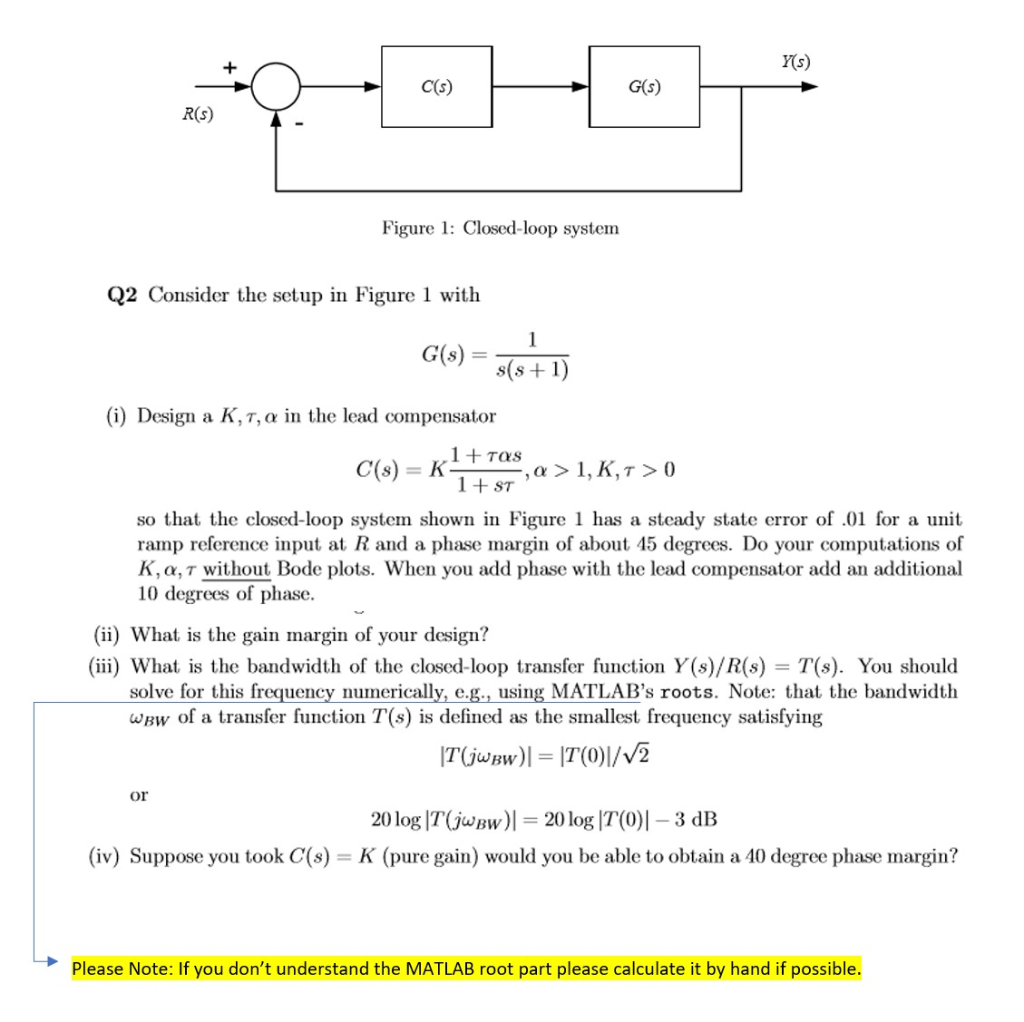Y(s) C(s) G(s) R(S) Figure 1: Closed-loop system Q2 Consider the setup in Figure 1 with S s1 (i) Design a K,τ, α in the lead compensator 1TOS so that the closed-loop system shown in Figure 1 has a steady state error of.0 for a unit ramp reference input at R and a phase margin of about 45 degrees K, α, τ without Bode plots. When you add phase with the lead compensator add an additional 10 degrees of phase....

• ### Problem 3. For the above feedback system, the bode diagram of the stable open-loop transfer function...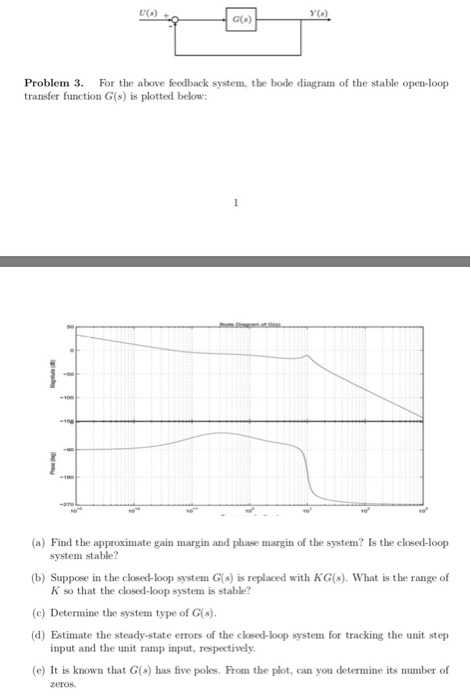Problem 3. For the above feedback system, the bode diagram of the stable open-loop transfer function G(s) is plotted below: (a) Find the approximate gain margin and phase margin of the system? Is the closed-loop system stable? (b) Suppose in the closed-loop system (s) is replaced with KG(8). What is the range of K so that the closed-loop system is stable? (C) Determine the system type of G(s). (d) Estimate the steady-state errors of the closed-loop system for tracking the...

• ### please show steps 5. GH(s) is a minimum-phase system which has the Bode plot shown below. It is desired to increase the phase margin by 40 degrees and also increase the closed-loop system bandwidth....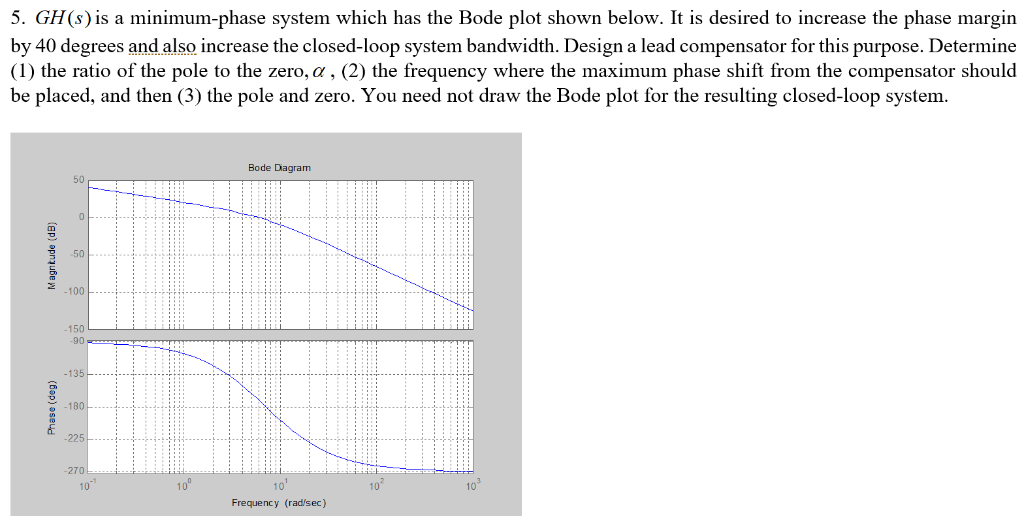please show steps 5. GH(s) is a minimum-phase system which has the Bode plot shown below. It is desired to increase the phase margin by 40 degrees and also increase the closed-loop system bandwidth. Design a lead compensator for this purpose. Determine (1) the ratio of the pole to the zero, α , (2) the frequency where the maximum phase shift from the compensator should be placed, and then (3) the pole and zero. You need not draw the Bode...

• ### P4) Consider a system with open loop transfer function of G(s) ? a) Sketch the Bode...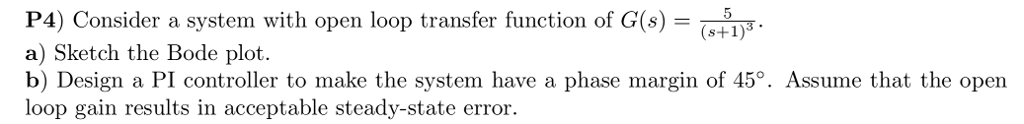P4) Consider a system with open loop transfer function of G(s) ? a) Sketch the Bode plot. b) Design a PI controller to make the system have a phase margin of 45°. Assume that the open loop s+1)3 gain results in acceptable steady-state error

• ### 5. The open loop transfer function of a control system is s(1 +0.5s)(1 0.67s) Draw a Bode diagram...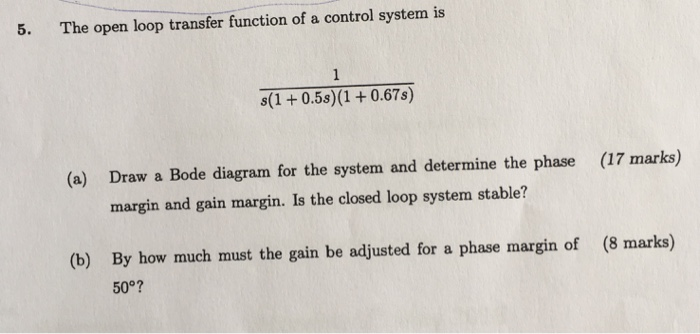5. The open loop transfer function of a control system is s(1 +0.5s)(1 0.67s) Draw a Bode diagram for the system and determine the phase margin and gain margin. Is the closed loop system stable? (a) (17 marks) (b) By how much must the gain be adjusted for a phase margin of 50°? (8 marks) 5. The open loop transfer function of a control system is s(1 +0.5s)(1 0.67s) Draw a Bode diagram for the system and determine the phase...

• ### Consider the transfer function of a DC motor given by G(s) = 1 / s(s+2) 3. Consider the transfer function of a DC motor...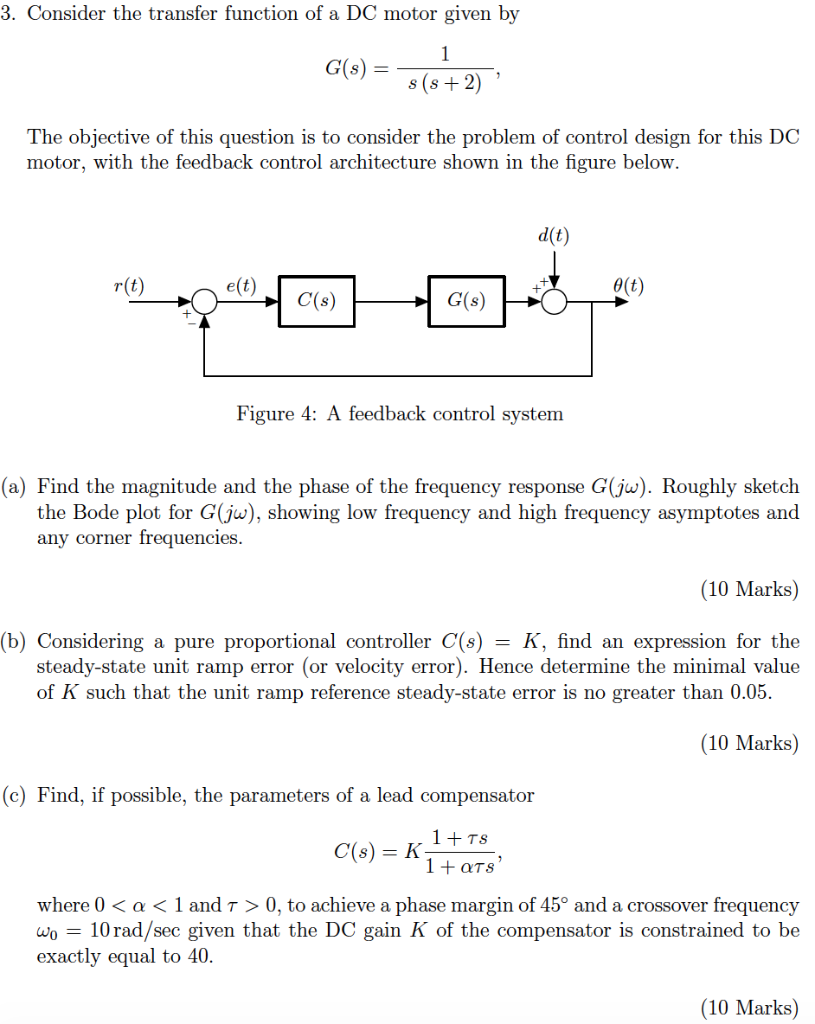Consider the transfer function of a DC motor given by G(s) = 1 / s(s+2) 3. Consider the transfer function of a DC motor given by 1 G(s) s (s2) The objective of this question is to consider the problem of control design for this DC motor, with the feedback control architecture shown in the figure below d(t r(t) e(t) e(t) C(s) G(s) Figure 4: A feedback control system (a) Find the magnitude and the phase of the frequency response...

• ### Determine the transfer function of the phase-lead compensator that can be used with the follow open-loop...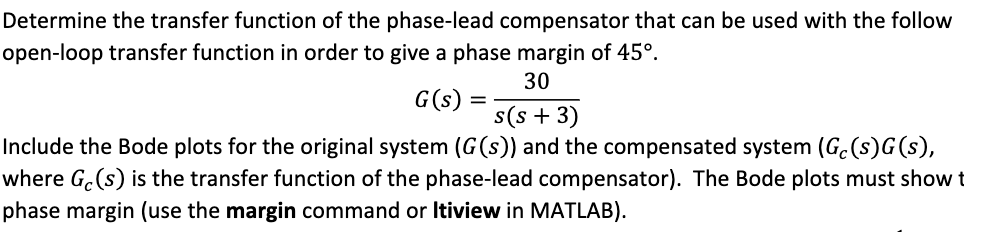Determine the transfer function of the phase-lead compensator that can be used with the follow open-loop transfer function in order to give a phase margin of 45° 30 G(s) = s(s+3) Include the Bode plots for the original system (G(s)) and the compensated system (Gc(s)G(s), where Gc(s) is the transfer function of the phase-lead compensator). The Bode plots must show t phase margin (use the margin command or Itiview in MATLAB).

• ### The transfer function of the given physical system is 2500 Gp(s)-T-1000 Part 3 1. Frequency response (a) Draw the bode...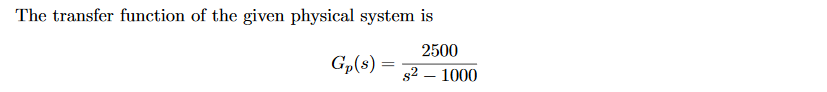The transfer function of the given physical system is 2500 Gp(s)-T-1000 Part 3 1. Frequency response (a) Draw the bode plot of open-loop transfer function when K (b) Use bode plot of open-loop transfer function to determine the type of system (do not use transfer function) (c) For what input the system will have constant steady-state error (d) for the unit input in item (c) calculate the constant steady-state error.(Use bode plot to calculate the error.) (e) Design a lead...